JEE  >  Test: Single Correct MCQs: States of Matter | JEE Advanced

# Test: Single Correct MCQs: States of Matter | JEE Advanced

Test Description

## 35 Questions MCQ Test Chemistry for JEE | Test: Single Correct MCQs: States of Matter | JEE Advanced

Test: Single Correct MCQs: States of Matter | JEE Advanced for JEE 2023 is part of Chemistry for JEE preparation. The Test: Single Correct MCQs: States of Matter | JEE Advanced questions and answers have been prepared according to the JEE exam syllabus.The Test: Single Correct MCQs: States of Matter | JEE Advanced MCQs are made for JEE 2023 Exam. Find important definitions, questions, notes, meanings, examples, exercises, MCQs and online tests for Test: Single Correct MCQs: States of Matter | JEE Advanced below.
Solutions of Test: Single Correct MCQs: States of Matter | JEE Advanced questions in English are available as part of our Chemistry for JEE for JEE & Test: Single Correct MCQs: States of Matter | JEE Advanced solutions in Hindi for Chemistry for JEE course. Download more important topics, notes, lectures and mock test series for JEE Exam by signing up for free. Attempt Test: Single Correct MCQs: States of Matter | JEE Advanced | 35 questions in 70 minutes | Mock test for JEE preparation | Free important questions MCQ to study Chemistry for JEE for JEE Exam | Download free PDF with solutions
 1 Crore+ students have signed up on EduRev. Have you?
Test: Single Correct MCQs: States of Matter | JEE Advanced - Question 1

### Equal weights of methane and oxygen are mixed in an empty container at 25ºC. The fraction of the total pressure exerted by oxygen is (1981 - 1 Mark)

Detailed Solution for Test: Single Correct MCQs: States of Matter | JEE Advanced - Question 1

TIPS/Formulae : Mole fraction of O2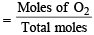Partial pressure of O2 = Mole fraction of O2

Mole fraction of O2 =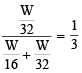Test: Single Correct MCQs: States of Matter | JEE Advanced - Question 2

### The temperature at which a real gas obeys the ideal gas laws over a wide range of pressure is (1981 - 1 Mark)

Detailed Solution for Test: Single Correct MCQs: States of Matter | JEE Advanced - Question 2

The temperature at which a real gas behaves like an ideal gas is called Boyle’s temperature or Boyle’s point.

Test: Single Correct MCQs: States of Matter | JEE Advanced - Question 3

### The ratio of root mean square velocity to average velocity of a gas molecule at a particular temperature is (1981 - 1 Mark)

Detailed Solution for Test: Single Correct MCQs: States of Matter | JEE Advanced - Question 3

Urms : Uav =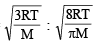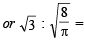= 1.086 : 1

Test: Single Correct MCQs: States of Matter | JEE Advanced - Question 4

Helium atom is two times heavier than a hydrogen molecule.At 298 K, the average kinetic energy of a helium atom is

(1982 - 1 Mark)

Detailed Solution for Test: Single Correct MCQs: States of Matter | JEE Advanced - Question 4

Average kinetic energy depends only on temperature and does not depend upon the nature of the gas. (∵ K.E. = 3/2 KT)

Test: Single Correct MCQs: States of Matter | JEE Advanced - Question 5

Equal weights of methane and hydrogen are mixed in an empty container at 25ºC. The fraction of the total pressure exerted by hydrogen is : (1984 - 1 Mark)

Detailed Solution for Test: Single Correct MCQs: States of Matter | JEE Advanced - Question 5

Pressure exerted by hydrogen will be proportional to its mole fraction.
Mole fraction of H2 =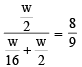Test: Single Correct MCQs: States of Matter | JEE Advanced - Question 6

Rate of diffusion of a gas is : (1985 - 1 Mark)

Detailed Solution for Test: Single Correct MCQs: States of Matter | JEE Advanced - Question 6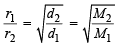Test: Single Correct MCQs: States of Matter | JEE Advanced - Question 7

The average velocity of an ideal gas molecule at 27ºC is 0.3 m/sec. The average velocity at 927ºC will be: (1986 - 1 Mark)

Detailed Solution for Test: Single Correct MCQs: States of Matter | JEE Advanced - Question 7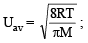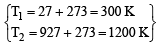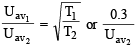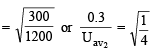or Uav2 = 0.6 m/sec.

Test: Single Correct MCQs: States of Matter | JEE Advanced - Question 8

In van der Waals equation of state for a non-ideal gas, the term that accounts for intermolecular forces is (1988 - 1 Mark)

Detailed Solution for Test: Single Correct MCQs: States of Matter | JEE Advanced - Question 8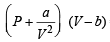= RTT; Here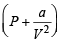represents
the intermolecular forces.

Test: Single Correct MCQs: States of Matter | JEE Advanced - Question 9

A bottle of dry ammonia and a bottle of dry hydrogen chloride connected through a long tube are opened simultaneously at both ends the white ammonium chloride ring first formed will be (1988 - 1 Mark)

Detailed Solution for Test: Single Correct MCQs: States of Matter | JEE Advanced - Question 9

TIPS/Formulae :

Rate of diffusion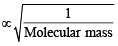∵ Molecular mass of HCl > molecular mass of NH3

∴ HCl diffuses at slower rate and white ammonium chloride is first formed near HCl bottle.

Test: Single Correct MCQs: States of Matter | JEE Advanced - Question 10

The values of van der Waals constant ‘a’ for the gases O2, N2, NH3 and CH4 are 1.360, 1.390, 4.170 and 2.253 L2 atm mol–2 respectively. The gas which can most easily be liquified is : (1989 - 1 Mark)

Detailed Solution for Test: Single Correct MCQs: States of Matter | JEE Advanced - Question 10

‘a’ is directly related to forces of attraction. Hence greater the value of ‘a’, more easily the gas is liquified.

Test: Single Correct MCQs: States of Matter | JEE Advanced - Question 11

The density of neon will be highest at (1990 - 1 Mark)

Detailed Solution for Test: Single Correct MCQs: States of Matter | JEE Advanced - Question 11

TIPS/Formulae :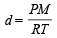It means density of gas is directly proportional to pressure and inversely proportional to temperature.
Density of neon will be maximum at highest pressure and lowest temperature.

Test: Single Correct MCQs: States of Matter | JEE Advanced - Question 12

The rate of diffusion of methane at a given temperature is twice that of a gas X. The molecular weight of X is (1990 - 1 Mark)

Detailed Solution for Test: Single Correct MCQs: States of Matter | JEE Advanced - Question 12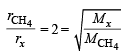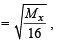or    Mx = 64

Test: Single Correct MCQs: States of Matter | JEE Advanced - Question 13

According to kinetic theory of gases, for a diatomic molecule (1991 - 1 Mark)

Detailed Solution for Test: Single Correct MCQs: States of Matter | JEE Advanced - Question 13

Pressure exerted by the gas,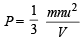...(1)

Here, u = root mean square velocity m = mass of a molecule, n = No. of molecules of the gas Hence (a) & (b) are clearly wrong.
Again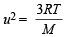[explained from (1)]

Here, M = Molecular wt. of the gas;

Hence (c) is wrong

Further, Average K.E. =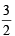KT;  Hence (d) is true.

Test: Single Correct MCQs: States of Matter | JEE Advanced - Question 14

At constant volume, for a fixed number of moles of a gas the pressure of the gas increases with rise in temperature due to (1992 - 1 Mark)

Detailed Solution for Test: Single Correct MCQs: States of Matter | JEE Advanced - Question 14

Due to increase in the temperature, the kinetic energy of the gas molecules increases resulting in an increase in average molecular speed. The molecules are bombarded to the walls of the container with a greater velocity resulting in an increase in pressure.

Test: Single Correct MCQs: States of Matter | JEE Advanced - Question 15

Longest mean free path stands for : (1995S)

Detailed Solution for Test: Single Correct MCQs: States of Matter | JEE Advanced - Question 15

The mean free path,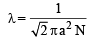or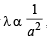where a = molecular diameter

∴ Smaller the molecular diameter, longer the mean free path. Hence H2 is the answer.

Test: Single Correct MCQs: States of Matter | JEE Advanced - Question 16

Arrange the van der Waals constant for the gases : (1995S)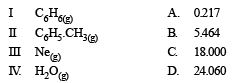Detailed Solution for Test: Single Correct MCQs: States of Matter | JEE Advanced - Question 16

NOTE : The value of ‘a’ indicates the magnitude of attractive forces between gas molecules.
Value of ‘a’ µ size of molecule.
∴ inert gas will have minimum value of ‘a’ followed by H2O, C6H6 and C6H5CH3

Test: Single Correct MCQs: States of Matter | JEE Advanced - Question 17

The ratio between the root mean square speed of H2 at 50 K and that of O2 at 800 K is, (1996 - 1 Mark)

Detailed Solution for Test: Single Correct MCQs: States of Matter | JEE Advanced - Question 17

The expression of root mean square speed is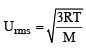Hence,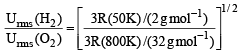= 1

Test: Single Correct MCQs: States of Matter | JEE Advanced - Question 18

X mL of H2 gas effuses through a hole in a container in 5 seconds. The time taken for the effusion of the same volume of the gas specified below under identical conditions is : (1996 - 1 Mark)

Detailed Solution for Test: Single Correct MCQs: States of Matter | JEE Advanced - Question 18

Under identical conditions,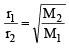As rate of diffusion is also inversely proportional to time, we will have,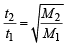(a) Thus,  For He,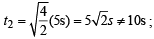(b) For O2, t2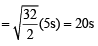(c) For CO, t2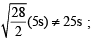(d) For CO2,  t2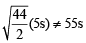Test: Single Correct MCQs: States of Matter | JEE Advanced - Question 19

One mole of N2O4(g) at 300 K is kept in a closed container under one atmosphere. It is heated to 600 K when 20% by mass of N2O4 (g) decomposes to NO2(g).The resultant pressure is : (1996 - 1 Mark)

Detailed Solution for Test: Single Correct MCQs: States of Matter | JEE Advanced - Question 19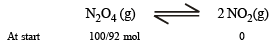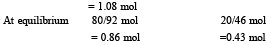According to ideal gas equation, at two conditions At 300 K;
P0V = n0RT0            1 × V = 1.08 ×R × 300                   …(i)
At 600 K;
P1V = n1RT1 P1× V = (0.86 +0.43) × R × 600      …(ii)
Divide (ii) by (i),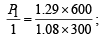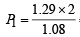= 2.38 atm.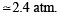Test: Single Correct MCQs: States of Matter | JEE Advanced - Question 20

The compressibility factor for an ideal gas is (1997 - 1 Mark)

Detailed Solution for Test: Single Correct MCQs: States of Matter | JEE Advanced - Question 20

The compressibility factor of a gas is defined asFor an ideal gas, pVm = RT. Hence Z = 1

Test: Single Correct MCQs: States of Matter | JEE Advanced - Question 21

A gas will  approach ideal behaviour at (1999 - 2 Marks)

Detailed Solution for Test: Single Correct MCQs: States of Matter | JEE Advanced - Question 21

For an ideal-gas behaviour, the molecules of a gas should be far apart. The factors favouring this condition are high temperature and low pressure.

Test: Single Correct MCQs: States of Matter | JEE Advanced - Question 22

The rms velocity of hydrogen is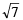times the rms velocity of nitrogen. If T is the temperature of the gas, then (2000S)

Detailed Solution for Test: Single Correct MCQs: States of Matter | JEE Advanced - Question 22

TIPS/Formulae :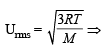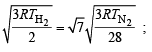∴ TN2= 2TH2 or TN2 >TH2

Test: Single Correct MCQs: States of Matter | JEE Advanced - Question 23

The compressibility of a gas is less than unity at STP.Therefore, (2000S)

Detailed Solution for Test: Single Correct MCQs: States of Matter | JEE Advanced - Question 23

(PV)Observed / (PV)Ideal < 1

⇒ Vobs < Videal, Vobs < 22.4 litre.

Test: Single Correct MCQs: States of Matter | JEE Advanced - Question 24

At 100°C and 1 atm, if the density of liquid water is 1.0 g cm–3 and that of water vapour is 0.0006 g cm–3, then the volume occupied by water molecules in 1 litre of steam at that temperature is (2000S)

Detailed Solution for Test: Single Correct MCQs: States of Matter | JEE Advanced - Question 24

Mass of 1 L of vapour = volume × density
= 1000 × 0.0006 = 0.6 g

V of liquid water =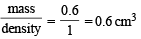Test: Single Correct MCQs: States of Matter | JEE Advanced - Question 25

The root mean square velocity of an ideal gas at constant pressure  varies with density (d) as (2001S)

Detailed Solution for Test: Single Correct MCQs: States of Matter | JEE Advanced - Question 25

URMS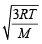Using ideal gas equation,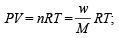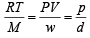where d is the
density of the gas

∴  URMS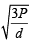at constant pressure,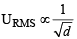Test: Single Correct MCQs: States of Matter | JEE Advanced - Question 26

Which of the following volume (V ) - temperature (T ) plots represents the behaviour of one mole of an ideal gas at one atmospheric pressure ? (2002S)

Detailed Solution for Test: Single Correct MCQs: States of Matter | JEE Advanced - Question 26

TIPS/Formulae : Find the volume by either
V = RT/P (PV = RT) or P1V1 = P2V2 and and match it with the values given in graph to find correct answer.
Volume of 1 mole of an ideal gas at 273 K and 1 atm is 22.4 L and that at 373 K and 1 atm pressure is calculated as ;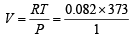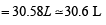Test: Single Correct MCQs: States of Matter | JEE Advanced - Question 27

When the temperature is increased, surface tension of water (2002S)

Detailed Solution for Test: Single Correct MCQs: States of Matter | JEE Advanced - Question 27

Upon increase of temperature the internal energy of water or any system increases resulting in decrease in intermolecular force and hence decrease in surface tension. Surface tension decreases with increase in mobility due to increase in temperature.

Test: Single Correct MCQs: States of Matter | JEE Advanced - Question 28

Positive deviation from ideal behaviour takes place because of(2003S)

Detailed Solution for Test: Single Correct MCQs: States of Matter | JEE Advanced - Question 28

For positive deviation: PV = nRT + nPb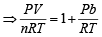Thus, the factor nPb is responsible for increasing the PV value,  above ideal value. b is actually the effective volume of molecule. So, it is the finite size of molecules that leads to the origin of b and hence positive deviation at high pressure.

Test: Single Correct MCQs: States of Matter | JEE Advanced - Question 29

The root mean square velocity of one mole of a monoatomic gas having molar mass M is ur.m.s.. The relation between the average kinetic energy (E) of the gas and ur.m.s. is (2004S)

Detailed Solution for Test: Single Correct MCQs: States of Matter | JEE Advanced - Question 29

Average KE = E =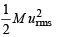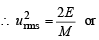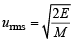Test: Single Correct MCQs: States of Matter | JEE Advanced - Question 30

The ratio of the rate of diffusion of helium and methane under identical condition of pressure and temperature will be(2005S)

Detailed Solution for Test: Single Correct MCQs: States of Matter | JEE Advanced - Question 30

TIPS/Formulae : Use Grahams’ law of diffusion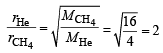Test: Single Correct MCQs: States of Matter | JEE Advanced - Question 31

When one mole of monoatomic ideal gas at T K undergoes adiabatic change under a constant external pressure of 1 atm volume changes from 1 litre to 2 litre. The final temperature in Kelvin would be (2005S)

Detailed Solution for Test: Single Correct MCQs: States of Matter | JEE Advanced - Question 31

TVγ-1  = Constant (Q change is adiabatic) 1
T1V1γ-1= T2V2γ-1

For monoatomic gas γ =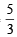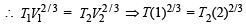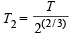Hence A is correct.

Test: Single Correct MCQs: States of Matter | JEE Advanced - Question 32

A mono-atomic ideal gas undergoes a process in which the ratio of P to V at any instant is constant and equals to 1.What is the molar heat capacity of the gas

Detailed Solution for Test: Single Correct MCQs: States of Matter | JEE Advanced - Question 32

In general, the molar heat capacity for any process is given by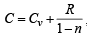when PVn = constant

Here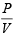=   i.e. PV–1 = constant

For monoatomic gas,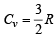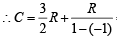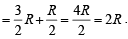Test: Single Correct MCQs: States of Matter | JEE Advanced - Question 33

The term that corrects for the attractive forces present in a real gas in the van der Waals equation is

Detailed Solution for Test: Single Correct MCQs: States of Matter | JEE Advanced - Question 33

Correction factor for attractive force for n moles of real gas is given by the term mentioned in (b).

Test: Single Correct MCQs: States of Matter | JEE Advanced - Question 34

For one mole of a van der Waal’s gas when b = 0 and T = 300 K, the PV vs, 1/V plot is shown below. The value of the van der Waal’s constant a (atm. liter2 mol–2) is : (2012)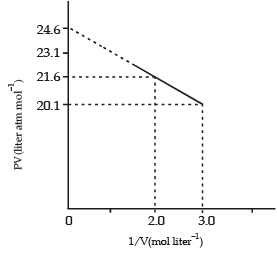Detailed Solution for Test: Single Correct MCQs: States of Matter | JEE Advanced - Question 34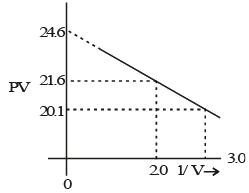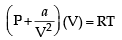PV + a/V = RT; PV = RT – a(V)

y = RT – a(x)

So,  slope = a =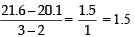Test: Single Correct MCQs: States of Matter | JEE Advanced - Question 35

The qualitative sketch es I, II and III given below show the variation of surface tension with molar concentration of three different aqueous solutions of KCl, CH3OH and CH3(CH2)11OSO3 Na+ at room temperature. The correct assignment of the sketches is (JEE Adv. 2016)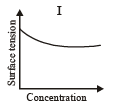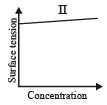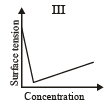Detailed Solution for Test: Single Correct MCQs: States of Matter | JEE Advanced - Question 35
• A solution of  CH3OH and water sh ows positive deviation from Raoult's law, it means by adding CH3OH intermolecular force of attraction decreases and hence surface tension decreases.
• By adding KCl in water, inter molecular force of attraction bit increases, so surface tension increases by small value.
• By adding surfactant like CH3(CH2)11OSO3Na+, surface tension decreases rapidly and after forming micelle it slightly increases.

## Chemistry for JEE

322 videos|586 docs|368 tests
 Use Code STAYHOME200 and get INR 200 additional OFF Use Coupon Code
Information about Test: Single Correct MCQs: States of Matter | JEE Advanced Page
In this test you can find the Exam questions for Test: Single Correct MCQs: States of Matter | JEE Advanced solved & explained in the simplest way possible. Besides giving Questions and answers for Test: Single Correct MCQs: States of Matter | JEE Advanced, EduRev gives you an ample number of Online tests for practice

## Chemistry for JEE

322 videos|586 docs|368 tests